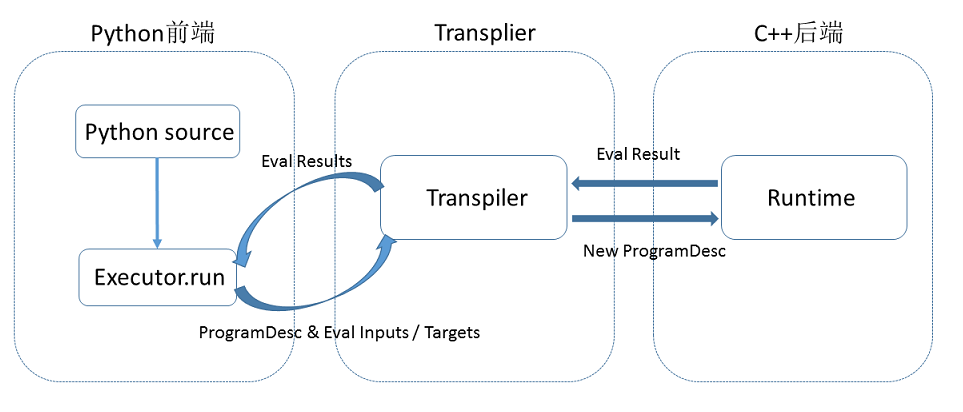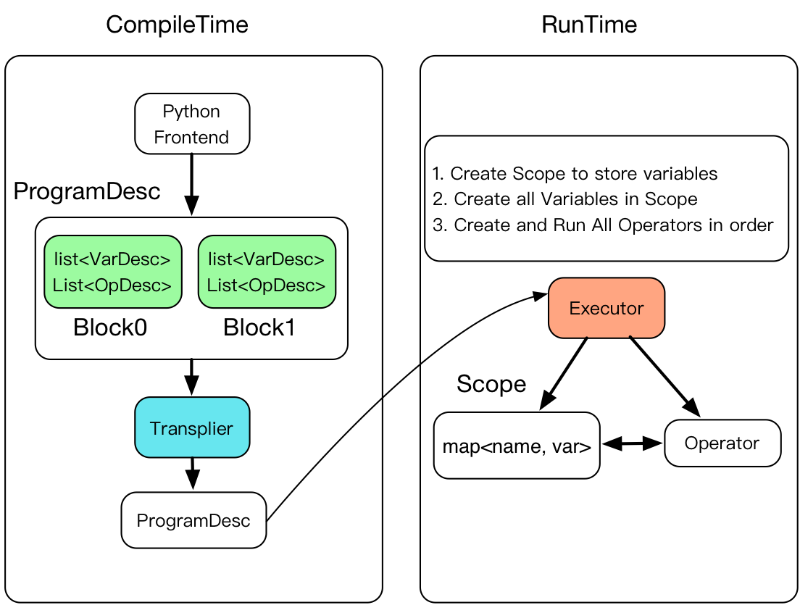设计思想¶

简介¶

• Fluid 内部的执行流程

• Program 如何描述模型

• Executor 如何执行运算

1. Fluid内部执行流程¶

Fluid使用一种编译器式的执行流程，分为编译时和运行时两个部分，具体包括：编译器定义 Program ，创建Executor 运行 Program 。1. 编译时，用户编写一段python程序，通过调用 Fluid 提供的算子，向一段 Program 中添加变量（Tensor）以及对变量的操作（Operators 或者 Layers）。用户只需要描述核心的前向计算，不需要关心反向计算、分布式下以及异构设备下如何计算。

2. 原始的 Program 在平台内部转换为中间描述语言： ProgramDesc

3. 编译期最重要的一个功能模块是 TranspilerTranspiler 接受一段 ProgramDesc ，输出一段变化后的 ProgramDesc ，作为后端 Executor 最终需要执行的 Fluid Program

4. 后端 Executor 接受 Transpiler 输出的这段 Program ，依次执行其中的 Operator（可以类比为程序语言中的指令），在执行过程中会为 Operator 创建所需的输入输出并进行管理。

2. Program设计思想¶

1. fluid.default_startup_program：定义了创建模型参数，输入输出，以及模型中可学习参数的初始化等各种操作

default_startup_program 可以由框架自动生成，使用时无需显示地创建

1. fluid.default_main_program ：定义了神经网络模型，前向反向计算，以及优化算法对网络中可学习参数的更新

Programs and Blocks¶

Fluid 的 Program 的基本结构是一些嵌套 blocks，形式上类似一段 C++ 或 Java 程序。

blocks中包含：

• 本地变量的定义

• 一系列的operator

block的概念与通用程序一致，例如在下列这段C++代码中包含三个block：

int main(){ //block 0
int i = 0;
if (i<10){ //block 1
for (int j=0;j<10;j++){ //block 2
}
}
return 0;
}

import paddle.fluid as fluid  # block 0

limit = fluid.layers.fill_constant_batch_size_like(
input=label, dtype='int64', shape=, value=5.0)
cond = fluid.layers.less_than(x=label, y=limit)

ie = fluid.layers.IfElse(cond)
with ie.true_block(): # block 1
true_image = ie.input(image)
hidden = fluid.layers.fc(input=true_image, size=100, act='tanh')
prob = fluid.layers.fc(input=hidden, size=10, act='softmax')
ie.output(prob)

with ie.false_block(): # block 2
false_image = ie.input(image)
hidden = fluid.layers.fc(
input=false_image, size=200, act='tanh')
prob = fluid.layers.fc(input=hidden, size=10, act='softmax')
ie.output(prob)

prob = ie()

BlockDesc and ProgramDesc¶

BlockDesc中包含本地变量的定义 vars，和一系列的operatorops

message BlockDesc {
required int32 parent = 1;
repeated VarDesc vars = 2;
repeated OpDesc ops = 3;
}

parent ID表示父块，因此block中的操作符可以引用本地定义的变量，也可以引用祖先块中定义的变量。

Program 中的每层 block 都被压平并存储在数组中。blocks ID是这个数组中块的索引。

message ProgramDesc {
repeated BlockDesc blocks = 1;
}

使用Blocks的Operator¶

[Programs and Blocks](#Programs and Blocks)的例子中，IfElseOp这个Operator包含了两个block——true分支和false分支。

message OpDesc {
AttrDesc attrs = 1;
...
}

message AttrDesc {
required string name = 1;

enum AttrType {
INT = 1,
STRING = 2,
...
BLOCK = ...
}
required AttrType type = 2;

optional int32 block = 10; // when type == BLOCK
...
}

3. Executor设计思想¶

Executor 在运行时将接受一个ProgramDesc、一个block_id和一个ScopeProgramDescblock的列表，每一项包含block中所有参数和operatorprotobuf定义；block_id指定入口块；Scope是所有变量实例的容器。1. Executor 为每一个block创建一个Scope，Block是可嵌套的，因此Scope也是可嵌套的

2. 创建所有Scope中的变量

3. 按顺序创建并执行所有operator

Executor的C++实现代码如下：

class Executor{
public:
void Run(const ProgramDesc& pdesc,
Scope* scope,
int block_id) {
auto& block = pdesc.Block(block_id);

//创建所有变量
for (auto& var : block.AllVars())
scope->Var(Var->Name());
}

//创建OP并按顺序执行
for (auto& op_desc : block.AllOps()){
auto op = CreateOp(*op_desc);
op->Run(*local_scope, place_);
}
}；

Fluid中使用fluid.Executor(place)创建Executor，place属性由用户定义，代表程序将在哪里执行。

cpu=fluid.CPUPlace()
exe = fluid.Executor(cpu)

Fluid使用Executor.run来运行程序。定义中通过Feed映射获取数据，通过fetch_list获取结果：

...
x = numpy.random.random(size=(10, 1)).astype('float32')
outs = exe.run(
feed={'X': x},
fetch_list=[loss.name])

代码实例¶

#加载函数库
import paddle.fluid as fluid #block 0
import numpy

#定义数据
train_data=numpy.array([[1.0],[2.0],[3.0],[4.0]]).astype('float32')
y_true = numpy.array([[2.0],[4.0],[6.0],[8.0]]).astype('float32')
#定义网络
x = fluid.layers.data(name="x",shape=,dtype='float32')
y = fluid.layers.data(name="y",shape=,dtype='float32')
y_predict = fluid.layers.fc(input=x,size=1,act=None)
#定义损失函数
cost = fluid.layers.square_error_cost(input=y_predict,label=y)
avg_cost = fluid.layers.mean(cost)
#定义优化方法
sgd_optimizer = fluid.optimizer.SGD(learning_rate=0.01)
sgd_optimizer.minimize(avg_cost)

print(fluid.default_main_program().to_string(True))

blocks {
idx: 0
parent_idx: -1
vars {
name: "mean_1.tmp_0"
type {
type: LOD_TENSOR
lod_tensor {
tensor {
data_type: FP32
dims: 1
}
}
}
persistable: false
}
vars {
name: "square_error_cost_1.tmp_1"
type {
type: LOD_TENSOR
lod_tensor {
tensor {
data_type: FP32
dims: -1
dims: 1
}
lod_level: 0
}
}
persistable: false
}
vars {
name: "square_error_cost_1.tmp_0"
type {
type: LOD_TENSOR
lod_tensor {
tensor {
data_type: FP32
dims: -1
dims: 1
}
lod_level: 0
}
}
persistable: false
...

BlockDesc中包含定义的 vars 和一系列的 ops，以输入x为例，python代码中定义 x 是一个数据类型为”float 32”的1维数据：

x = fluid.layers.data(name="x",shape=,dtype='float32')

vars {
name: "x"
type {
type: LOD_TENSOR
lod_tensor {
tensor {
data_type: FP32
dims: -1
dims: 1
}
lod_level: 0
}
}
persistable: false

dims表示数据的维度，这里表示 x 的维度为[-1,1]，其中-1是batch的维度，无法确定具体数值时，Fluid 自动用 -1 占位。

Fluid使用Executor来执行网络训练，Executor运行细节请参考Executor设计思想的介绍。作为使用者，实际并不需要了解内部机制。

#在CPU内执行训练
cpu = fluid.CPUPlace()
#创建Executor
exe = fluid.Executor(cpu)

Fluid使用Executor.run来运行一段Program。

#参数初始化
exe.run(fluid.default_startup_program())

#开始训练
outs = exe.run(
feed={'x':train_data,'y':y_true},
fetch_list=[y_predict.name,avg_cost.name])

[array([[1.5248038],
[3.0496075],
[4.5744114],
[6.099215 ]], dtype=float32), array([1.6935859], dtype=float32)]